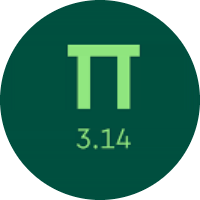# Solving Geometric Measurement Problems

In this quiz, you will get to know many concepts about Geometric measurement. You will learn about the perimeter and area of the different triangles, squares, rectangles, trapezoids, compound figures, cubes, cylinders, cones, and spheres.

Start Quiz

A rectangle has a perimeter of 45.6 centimeters and a base of 12 centimeters. What is the height?

9.8 cm

12 cm

10.8 cm

15.8 cm

Find the area.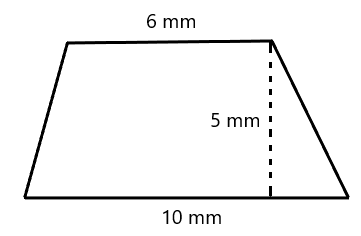60

40

50

30

The area of a postcard is 15 square inches. The perimeter is 16 inches. What are the dimensions of the postcard?

4 inches by 5 inches

2 inches by 5 inches

2 inches by 4 inches

3 inches by 5 inches

A coffee mug has a radius of 4 centimeters. What is the mug's circumference?

Use 3.14 for ​𝜋.

25.12

18.64

23.5

12.45

What is the area of the shaded region?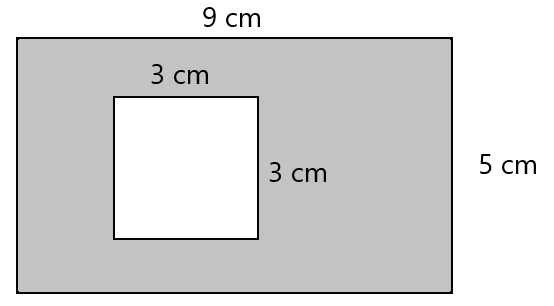9

45

15

36

What is the surface area of the cube if all sides are equal and measure 3 inches?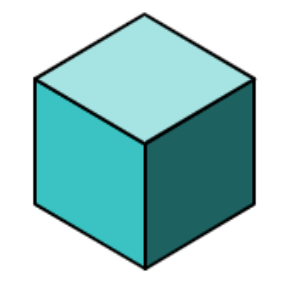4

24

12

36

A cylinder has a height of 12 feet and a radius of 11 feet. What is its volume? Use ​𝜋 ≈ 3.14 and round your answer to the nearest hundredth.

4334.87

3245.45

4559.28

3487.35

A cone has a height of 12 millimeters and a radius of 6 millimeters. What is its volume?

Use ​𝜋 ≈ 3.14 and round your answer to the nearest hundredth.

234.98

435.87

345.90

452.16

What is the volume of this sphere?

Use ​𝜋 ≈ 3.14 and round your answer to the nearest hundredth.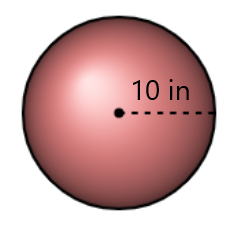4186.67

4356.33

3834.67

Look at this square: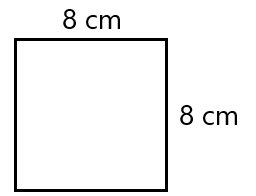If the side lengths are doubled, then which of the following statements about its area will be true?

The new area will be 8 times the old area.

The new area will be 2 times the old area.

The new area will be 14 of the old area.

The new area will be 4 times the old area.

Quiz/Test Summary
Title: Solving Geometric Measurement Problems
Questions: 10
Contributed by: